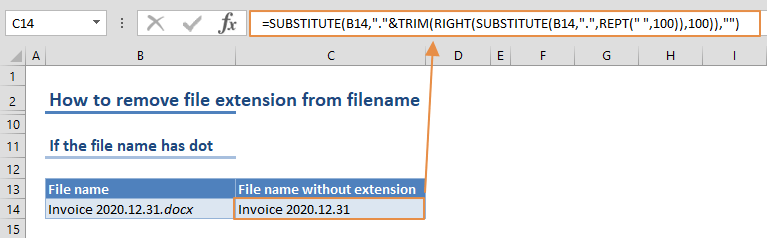If you want to remove file extension from full file names, first you need to locate the period (.) which separates the filename and the extension. This action is fairly easy to do if your file names do not contain any periods other than the extension separator. Otherwise, you might want to use a formula to locate the last period and remove the extension. In this guide, we are going to show you how to remove file extension from filename in two different ways.

## Simple formula for filenames without dots

If your file name doesn't contain any dot (.) characters except for the extension separator. This simple formula should work:

=LEFT(filename,FIND(".",filename)-1)

This formula has 2 steps:

1. FIND function locates the dot character (.) and returns its position as a number
2. LEFT function extracts the characters up to one less character where the period is

For example, for the string "Superman.png", the period is in the 9th position. To parse only "Superman", you need get the first 8th characters of the name. This is where the subtracting one operation comes in. =LEFT("Superman.png",8) returns "Superman".The downside of this approach is that the formula only looks for the first period. If you have multiple periods except for the extension separator, the formula will remove everything after the first period.

For example, if the text is "Invoice 2020.12.31.docx", the formula returns only the "Invoice 2020" part.### File names with dots

If the text you want to work with contains multiple periods, you need to specifically locate the last one separating the extension. Unfortunately, Excel doesn't have a dedicated function to begin a search from right-to-left. So, we're going to use a different approach here.

=SUBSTITUTE(filename,"."&TRIM(RIGHT(SUBSTITUTE(filename,".",REPT(" ",100)),100)),"")

Don't let the length of the formula intimidate you. Let's break it down:

• SUBSTITUTE(filename,".",REPT(" ",100)) replaces each period with 100 spaces. The number 100 is a big enough value to move the extension at the end to the remaining part of the string.
• TRIM and RIGHT functions return the right 100 characters and trims the name to get the extension from the end.
• Finally, the SUBSTITUTE function replaces a period and the extension string with an empty string ("").

Briefly, the formula finds the extension first, and then cuts the file name.